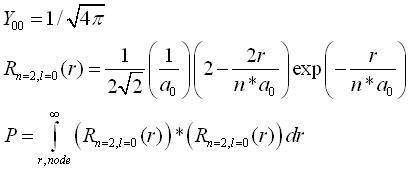# Wavefunction of a 2s hydrogen electron.

## Homework Statement

a)Write Complete wave function for an electron in a 2s orbital of hydrogen
b)find the probability that the electron is at a distance from the nucleus that is outside the radius of the node.
c)graph the radial distribution function for this system.

## Homework Equations

using quantum numbers of n=2 l=0 ml=0 ms=+/- (1/2)

Z = 1

eps = (8.854187816*10^(-12))/10000000000*Coulomb^2*Joule^(-1)*Angstrom^(-1)

me = 9.1093897*10^(-31) Kilogram

e = 1.602177338*10^(-19) Coulomb

h = 1.05457266*10^(-34) Joule*Second

a = 4*π*eps*h^2/(me*e^2)

R[r*Angstrom_] = (1\(2*2^(1/2)))*((Z/a)^(3/2))*(2 -
1/(2 p[r*Angstrom]))*Exp[-p[r*Angstrom]/4] (This is the Radial Wavefunc)

Y = (1/(2*π)^(1/2))*1*(2^(1/2)/2) (The complete roational wave func for theta and phi)

Λ = 1 (The spin wave function, is this right? since it has only one electron?)

## The Attempt at a Solution

I did the solution in mathematica, what i did was set R=0 and solved for r which came out with units of (J*s^2)/(Kg*Angstrom^2) which doesnt seem right to me shouldnt r be a unit of distance in general? Ecspecially at the node right?.
Btw the node came out to be 6.614715537095822*10^-22 (J*s^2)/(Kg*Angstrom^2)

So i took that node and integrated r^2*R^2(r) from 0 to the node, times the integral from zero to 2pi dPhi times the integral of zero to pi of Y^2sin(theta)dTheat

∫(0 to node) r*r*R(r)*R(r) dr * ∫(0 to 2pi) dPhi *∫(0 to pi) Y*Y*sin(Theta) dTheta

This gave me 0.0003155974/Angstrom (Shouldn't it be dimensionless?)

Thus giving me what i think is the probablity (i took away the unit) of the elecron being form within the radius to the node so i took this answer and minused it from 1 to get the probability of the electron being beyond the node which was 0.999684 which is very but for a 2s orbital it is kinda reasonable.

but when it comes to graphing, the radial wavefunction times r^2 times 4 pi (the radial distrubution function) gives me non machine sized numbers, or it gives underflow (the number is to small for the machine to realize its not zero)
any suggestions or tips? And am I doing this right? Thank ya'll for takin the time to read it!

malawi_glenn
Homework Helper
The spherical harmonics are normalised so you dont need to take accont for them when you do the integration.

I use these equations:a_0 is the Bohr radius, 0.5291 Å

I get r,node = 1,057 bohr radius

Probability to be outside the node = 0,064

I used a Texas Ti-83 for this (and one year old quantum mechanics knowledge)

#### Attachments

malawi_glenn
Homework Helper
∫(0 to node) r*r*R(r)*R(r) dr * ∫(0 to 2pi) dPhi *∫(0 to pi) Y*Y*sin(Theta) dTheta

This gave me 0.0003155974/Angstrom (Shouldn't it be dimensionless?)

Haven't you calculated the <r^2> ?

Are those commas on accident, o is that supposed to be periods? Because 559 Angstroms is way to much and if thats a 64, a probability has be between 0 and 1. So what ive done since i posted this is ive fixed all my units, i had forgotten to break down the Joules term, I got a node at radius .066 Angstrum and the same probability. Also your Y term is missing the Legendre Polynomial (the Theta term).

As for calculating r^2 and integrating using Phi and Theta, its part of the radial distribution function to distribute the probability around a sphere instead of just a singe direction. But i get the same probability doing it ur way as well, so i see how they are normalized.

Btw I have to do it in mathematica, which is good and bad, good because on the previous problem i had a determinant that was 2 pages long computer typed, then had to solve it. XD That woulda been horrible by hand. Bad because the program can be so frustrating sometimes.